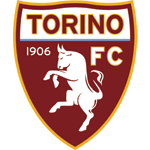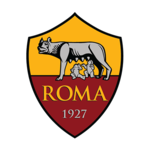# .css-adzqt3{display:inline-block;word-break:break-word;opacity:0;position:relative;-webkit-animation:animation-plwpox 0ms 0.4s forwards;animation:animation-plwpox 0ms 0.4s forwards;}.css-adzqt3::after{content:"";position:absolute;width:calc(100% + 14px);height:100%;top:0px;left:0px;background-color:var(--chakra-colors-light-white_100);-webkit-transform:scale3d(0, 1.05, 1);-moz-transform:scale3d(0, 1.05, 1);-ms-transform:scale3d(0, 1.05, 1);transform:scale3d(0, 1.05, 1);-webkit-animation:animation-f981je 250ms 0.15000000000000002s forwards step-end,animation-1ncn112 250ms 0.65s forwards step-end;animation:animation-f981je 250ms 0.15000000000000002s forwards step-end,animation-1ncn112 250ms 0.65s forwards step-end;}@-webkit-keyframes animation-1ncn112{0%{-webkit-transform:scale3d(0.75, 1.05, 1);-moz-transform:scale3d(0.75, 1.05, 1);-ms-transform:scale3d(0.75, 1.05, 1);transform:scale3d(0.75, 1.05, 1);transform-origin:top right;}44.4%{-webkit-transform:scale3d(0.25, 1.05, 1);-moz-transform:scale3d(0.25, 1.05, 1);-ms-transform:scale3d(0.25, 1.05, 1);transform:scale3d(0.25, 1.05, 1);transform-origin:top right;}100%{-webkit-transform:scale3d(0, 1.05, 1);-moz-transform:scale3d(0, 1.05, 1);-ms-transform:scale3d(0, 1.05, 1);transform:scale3d(0, 1.05, 1);transform-origin:top right;}}@keyframes animation-1ncn112{0%{-webkit-transform:scale3d(0.75, 1.05, 1);-moz-transform:scale3d(0.75, 1.05, 1);-ms-transform:scale3d(0.75, 1.05, 1);transform:scale3d(0.75, 1.05, 1);transform-origin:top right;}44.4%{-webkit-transform:scale3d(0.25, 1.05, 1);-moz-transform:scale3d(0.25, 1.05, 1);-ms-transform:scale3d(0.25, 1.05, 1);transform:scale3d(0.25, 1.05, 1);transform-origin:top right;}100%{-webkit-transform:scale3d(0, 1.05, 1);-moz-transform:scale3d(0, 1.05, 1);-ms-transform:scale3d(0, 1.05, 1);transform:scale3d(0, 1.05, 1);transform-origin:top right;}}@-webkit-keyframes animation-f981je{0%{-webkit-transform:scale3d(0, 1.05, 1);-moz-transform:scale3d(0, 1.05, 1);-ms-transform:scale3d(0, 1.05, 1);transform:scale3d(0, 1.05, 1);transform-origin:top left;}22.2%{-webkit-transform:scale3d(0.25, 1.05, 1);-moz-transform:scale3d(0.25, 1.05, 1);-ms-transform:scale3d(0.25, 1.05, 1);transform:scale3d(0.25, 1.05, 1);transform-origin:top left;}44.4%{-webkit-transform:scale3d(0.5, 1.05, 1);-moz-transform:scale3d(0.5, 1.05, 1);-ms-transform:scale3d(0.5, 1.05, 1);transform:scale3d(0.5, 1.05, 1);transform-origin:top left;}66.6%{-webkit-transform:scale3d(0.75, 1.05, 1);-moz-transform:scale3d(0.75, 1.05, 1);-ms-transform:scale3d(0.75, 1.05, 1);transform:scale3d(0.75, 1.05, 1);transform-origin:top left;}100%{-webkit-transform:scale3d(1, 1.05, 1);-moz-transform:scale3d(1, 1.05, 1);-ms-transform:scale3d(1, 1.05, 1);transform:scale3d(1, 1.05, 1);transform-origin:top left;}}@keyframes animation-f981je{0%{-webkit-transform:scale3d(0, 1.05, 1);-moz-transform:scale3d(0, 1.05, 1);-ms-transform:scale3d(0, 1.05, 1);transform:scale3d(0, 1.05, 1);transform-origin:top left;}22.2%{-webkit-transform:scale3d(0.25, 1.05, 1);-moz-transform:scale3d(0.25, 1.05, 1);-ms-transform:scale3d(0.25, 1.05, 1);transform:scale3d(0.25, 1.05, 1);transform-origin:top left;}44.4%{-webkit-transform:scale3d(0.5, 1.05, 1);-moz-transform:scale3d(0.5, 1.05, 1);-ms-transform:scale3d(0.5, 1.05, 1);transform:scale3d(0.5, 1.05, 1);transform-origin:top left;}66.6%{-webkit-transform:scale3d(0.75, 1.05, 1);-moz-transform:scale3d(0.75, 1.05, 1);-ms-transform:scale3d(0.75, 1.05, 1);transform:scale3d(0.75, 1.05, 1);transform-origin:top left;}100%{-webkit-transform:scale3d(1, 1.05, 1);-moz-transform:scale3d(1, 1.05, 1);-ms-transform:scale3d(1, 1.05, 1);transform:scale3d(1, 1.05, 1);transform-origin:top left;}}@-webkit-keyframes animation-plwpox{from{opacity:0;}to{opacity:1;}}@keyframes animation-plwpox{from{opacity:0;}to{opacity:1;}}SEASON• September 2023
• Serie A TIMEmpoli

Sep 24th 2023 — 10:30Inter
•Inter

Sep 27th 2023 — 18:45Sassuolo
•Salernitana

Sep 30th 2023 — 18:45Inter
• October 2023
• Champions LeagueInter

Oct 3rd 2023 — 19:00Benfica
• Serie A TIMInter

Oct 7th 2023 — 13:00Bologna
•Torino

Oct 21st 2023 — 16:00Inter
•Inter

Oct 29th 2023 — 17:00Roma

## Serie Astandings

G

W

D

L

GS

P

1Inter

4

4

0

0

13-1

12

2Milan

5

4

0

1

10-7

12

3Lecce

5

3

2

0

8-4

11

4Juventus

5

3

1

1

11-6

10

• Club

• Inter Store

Language